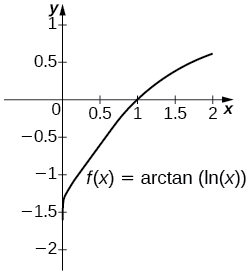$$\newcommand{\id}{\mathrm{id}}$$ $$\newcommand{\Span}{\mathrm{span}}$$ $$\newcommand{\kernel}{\mathrm{null}\,}$$ $$\newcommand{\range}{\mathrm{range}\,}$$ $$\newcommand{\RealPart}{\mathrm{Re}}$$ $$\newcommand{\ImaginaryPart}{\mathrm{Im}}$$ $$\newcommand{\Argument}{\mathrm{Arg}}$$ $$\newcommand{\norm}{\| #1 \|}$$ $$\newcommand{\inner}{\langle #1, #2 \rangle}$$ $$\newcommand{\Span}{\mathrm{span}}$$

# 5.7E: Exercises for Section 5.7

$$\newcommand{\vecs}{\overset { \rightharpoonup} {\mathbf{#1}} }$$ $$\newcommand{\vecd}{\overset{-\!-\!\rightharpoonup}{\vphantom{a}\smash {#1}}}$$$$\newcommand{\id}{\mathrm{id}}$$ $$\newcommand{\Span}{\mathrm{span}}$$ $$\newcommand{\kernel}{\mathrm{null}\,}$$ $$\newcommand{\range}{\mathrm{range}\,}$$ $$\newcommand{\RealPart}{\mathrm{Re}}$$ $$\newcommand{\ImaginaryPart}{\mathrm{Im}}$$ $$\newcommand{\Argument}{\mathrm{Arg}}$$ $$\newcommand{\norm}{\| #1 \|}$$ $$\newcommand{\inner}{\langle #1, #2 \rangle}$$ $$\newcommand{\Span}{\mathrm{span}}$$ $$\newcommand{\id}{\mathrm{id}}$$ $$\newcommand{\Span}{\mathrm{span}}$$ $$\newcommand{\kernel}{\mathrm{null}\,}$$ $$\newcommand{\range}{\mathrm{range}\,}$$ $$\newcommand{\RealPart}{\mathrm{Re}}$$ $$\newcommand{\ImaginaryPart}{\mathrm{Im}}$$ $$\newcommand{\Argument}{\mathrm{Arg}}$$ $$\newcommand{\norm}{\| #1 \|}$$ $$\newcommand{\inner}{\langle #1, #2 \rangle}$$ $$\newcommand{\Span}{\mathrm{span}}$$

In exercises 1 - 6, evaluate each integral in terms of an inverse trigonometric function.

1) $$\displaystyle ∫^{\sqrt{3}/2}_0\frac{dx}{\sqrt{1−x^2}}$$

$$\displaystyle ∫^{\sqrt{3}/2}_0\frac{dx}{\sqrt{1−x^2}} \quad = \quad \sin^{−1}x\bigg|^{\sqrt{3}/2}_0=\dfrac{π}{3}$$

2) $$\displaystyle ∫^{1/2}_{−1/2}\frac{dx}{\sqrt{1−x^2}}$$

3) $$\displaystyle ∫^1_{\sqrt{3}}\frac{dx}{\sqrt{1+x^2}}$$

$$\displaystyle ∫^1_{\sqrt{3}}\frac{dx}{\sqrt{1+x^2}} \quad = \quad \tan^{−1}x\bigg|^1_{\sqrt{3}}=−\dfrac{π}{12}$$

4) $$\displaystyle ∫^{\sqrt{3}}_{\frac{1}{\sqrt{3}}}\frac{dx}{1+x^2}$$

5) $$\displaystyle ∫^{\sqrt{2}}_1\frac{dx}{|x|\sqrt{x^2−1}}$$

$$\displaystyle ∫^{\sqrt{2}}_1\frac{dx}{|x|\sqrt{x^2−1}} \quad = \quad \sec^{−1}x\bigg|^{\sqrt{2}}_1=\dfrac{π}{4}$$

6) $$\displaystyle ∫^{\frac{2}{\sqrt{3}}}_1\frac{dx}{|x|\sqrt{x^2−1}}$$

In exercises 7 - 12, find each indefinite integral, using appropriate substitutions.

7) $$\displaystyle ∫\frac{dx}{\sqrt{9−x^2}}$$

$$\displaystyle ∫\frac{dx}{\sqrt{9−x^2}} \quad = \quad \sin^{−1}\left(\frac{x}{3}\right)+C$$

8) $$\displaystyle ∫\frac{dx}{\sqrt{1−16x^2}}$$

9) $$\displaystyle ∫\frac{dx}{9+x^2}$$

$$\displaystyle ∫\frac{dx}{9+x^2} \quad = \quad \frac{1}{3}\tan^{−1}\left(\frac{x}{3}\right)+C$$

10) $$\displaystyle ∫\frac{dx}{25+16x^2}$$

11) $$\displaystyle ∫\frac{dx}{x\sqrt{x^2−9}}$$

$$\displaystyle ∫\frac{dx}{x\sqrt{x^2−9}} \quad = \quad \frac{1}{3}\sec^{−1}\left(\frac{|x|}{3}\right)+C$$

12) $$\displaystyle ∫\frac{dx}{x\sqrt{4x^2−16}}$$

13) Explain the relationship $$\displaystyle −\cos^{−1}t+C=∫\frac{dt}{\sqrt{1−t^2}}=\sin^{−1}t+C.$$ Is it true, in general, that $$\cos^{−1}t=−\sin^{−1}t$$?

$$\cos(\frac{π}{2}−θ)=\sin θ.$$ So, $$\sin^{−1}t=\dfrac{π}{2}−\cos^{−1}t.$$ They differ by a constant.

14) Explain the relationship $$\displaystyle \sec^{−1}t+C=∫\frac{dt}{|t|\sqrt{t^2−1}}=−\csc^{−1}t+C.$$ Is it true, in general, that $$\sec^{−1}t=−\csc^{−1}t$$?

15) Explain what is wrong with the following integral: $$\displaystyle ∫^2_1\frac{dt}{\sqrt{1−t^2}}$$.

$$\sqrt{1−t^2}$$ is not defined as a real number when $$t>1$$.

16) Explain what is wrong with the following integral: $$\displaystyle ∫^1_{−1}\frac{dt}{|t|\sqrt{t^2−1}}$$.

$$\sqrt{t^2−1}$$ is not defined as a real number when $$-1 \lt t \lt 1$$, and the integrand is undefined when $$t = -1$$ or $$t = 1$$.

In exercises 17 - 20, solve for the antiderivative of $$f$$ with $$C=0$$, then use a calculator to graph $$f$$ and the antiderivative over the given interval $$[a,b]$$. Identify a value of $$C$$ such that adding $$C$$ to the antiderivative recovers the definite integral $$\displaystyle F(x)=∫^x_af(t)\,dt$$.

17) [T] $$\displaystyle ∫\frac{1}{\sqrt{9−x^2}}\,dx$$ over $$[−3,3]$$The antiderivative is $$\sin^{−1}(\frac{x}{3})+C$$. Taking $$C=\frac{π}{2}$$ recovers the definite integral.

18) [T] $$\displaystyle ∫\frac{9}{9+x^2}\,dx$$ over $$[−6,6]$$

19) [T] $$\displaystyle ∫\frac{\cos x}{4+\sin^2x}\,dx$$ over $$[−6,6]$$The antiderivative is $$\frac{1}{2}\tan^{−1}(\frac{\sin x}{2})+C$$. Taking $$C=\frac{1}{2}\tan^{−1}(\frac{\sin(6)}{2})$$ recovers the definite integral.

20) [T] $$\displaystyle ∫\frac{e^x}{1+e^{2x}}\,dx$$ over $$[−6,6]$$

In exercises 21 - 26, compute the antiderivative using appropriate substitutions.

21) $$\displaystyle ∫\frac{\sin^{−1}t}{\sqrt{1−t^2}}\,dt$$

$$\displaystyle ∫\frac{\sin^{−1}t\,dt}{\sqrt{1−t^2}} \quad = \quad \tfrac{1}{2}(\sin^{−1}t)^2+C$$

22) $$\displaystyle ∫\frac{dt}{\sin^{−1} t\sqrt{1−t^2}}$$

23) $$\displaystyle ∫\frac{\tan^{−1}(2t)}{1+4t^2}\,dt$$

$$\displaystyle ∫\frac{\tan^{−1}(2t)}{1+4t^2}\,dt \quad = \quad \frac{1}{4}(\tan^{−1}(2t))^2+C$$

24) $$\displaystyle ∫\frac{t\tan^{−1}(t^2)}{1+t^4}\,dt$$

25) $$\displaystyle ∫\frac{\sec^{−1}\left(\tfrac{t}{2}\right)}{|t|\sqrt{t^2−4}}\,dt$$

$$\displaystyle ∫\frac{\sec^{−1}\left(\tfrac{t}{2}\right)}{|t|\sqrt{t^2−4}}\,dt \quad = \quad \tfrac{1}{4}(\sec^{−1}\left(\tfrac{t}{2}\right))^2+C$$

26) $$\displaystyle ∫\frac{t\sec^{−1}(t^2)}{t^2\sqrt{t^4−1}}\,dt$$

In exercises 27 - 32, use a calculator to graph the antiderivative of $$f$$ with $$C=0$$ over the given interval $$[a,b].$$ Approximate a value of $$C$$, if possible, such that adding $$C$$ to the antiderivative gives the same value as the definite integral $$\displaystyle F(x)=∫^x_af(t)\,dt.$$

27) [T] $$\displaystyle ∫\frac{1}{x\sqrt{x^2−4}}\,dx$$ over $$[2,6]$$The antiderivative is $$\frac{1}{2}\sec^{−1}(\frac{x}{2})+C$$. Taking $$C=0$$ recovers the definite integral over $$[2,6]$$.

28) [T] $$\displaystyle ∫\frac{1}{(2x+2)\sqrt{x}}\,dx$$ over $$[0,6]$$

29) [T] $$\displaystyle ∫\frac{(\sin x+x\cos x)}{1+x^2\sin^2x\,dx}$$ over $$[−6,6]$$The general antiderivative is $$\tan^{−1}(x\sin x)+C$$. Taking $$C=−\tan^{−1}(6\sin(6))$$ recovers the definite integral.

30) [T] $$\displaystyle ∫\frac{2e^{−2x}}{\sqrt{1−e^{−4x}}}\,dx$$ over $$[0,2]$$

31) [T] $$\displaystyle ∫\frac{1}{x+x\ln 2x}$$ over $$[0,2]$$The general antiderivative is $$\tan^{−1}(\ln x)+C$$. Taking $$\displaystyle C=\tfrac{π}{2}=\lim_{t \to ∞}\tan^{−1} t$$ recovers the definite integral.

32) [T] $$\displaystyle ∫\frac{\sin^{−1}x}{\sqrt{1−x^2}}$$ over $$[−1,1]$$

In exercises 33 - 38, compute each integral using appropriate substitutions.

33) $$\displaystyle ∫\frac{e^x}{\sqrt{1−e^{2t}}}\,dt$$

$$\displaystyle ∫\frac{e^x}{\sqrt{1−e^{2t}}}\,dt \quad = \quad \sin^{−1}(e^t)+C$$

34) $$\displaystyle ∫\frac{e^t}{1+e^{2t}}\,dt$$

35) $$\displaystyle ∫\frac{dt}{t\sqrt{1−\ln^2t}}$$

$$\displaystyle ∫\frac{dt}{t\sqrt{1−\ln^2t}} \quad = \quad \sin^{−1}(\ln t)+C$$

36) $$\displaystyle ∫\frac{dt}{t(1+\ln^2t)}$$

37) $$\displaystyle ∫\frac{\cos^{−1}(2t)}{\sqrt{1−4t^2}}\,dt$$

$$\displaystyle ∫\frac{\cos^{−1}(2t)}{\sqrt{1−4t^2}}\,dt \quad = \quad −\frac{1}{2}(\cos^{−1}(2t))^2+C$$

38) $$\displaystyle ∫\frac{e^t\cos^{−1}(e^t)}{\sqrt{1−e^{2t}}}\,dt$$

In exercises 39 - 42, compute each definite integral.

39) $$\displaystyle ∫^{1/2}_0\frac{\tan(\sin^{−1}t)}{\sqrt{1−t^2}}\,dt$$

$$\displaystyle ∫^{1/2}_0\frac{\tan(\sin^{−1}t)}{\sqrt{1−t^2}}\,dt \quad = \quad \frac{1}{2}\ln\left(\frac{4}{3}\right)$$

40) $$\displaystyle ∫^{1/2}_{1/4}\frac{\tan(\cos^{−1}t)}{\sqrt{1−t^2}}\,dt$$

41) $$\displaystyle ∫^{1/2}_0\frac{\sin(\tan^{−1}t)}{1+t^2}\,dt$$

$$\displaystyle ∫^{1/2}_0\frac{\sin(\tan^{−1}t)}{1+t^2}\,dt \quad = \quad 1−\frac{2}{\sqrt{5}}$$

42) $$\displaystyle ∫^{1/2}_0\frac{\cos(\tan^{−1}t)}{1+t^2}\,dt$$

43) For $$A>0$$, compute $$\displaystyle I(A)=∫^{A}_{−A}\frac{dt}{1+t^2}$$ and evaluate $$\displaystyle \lim_{a→∞}I(A)$$, the area under the graph of $$\dfrac{1}{1+t^2}$$ on $$[−∞,∞]$$.

$$2\tan^{−1}(A)→π$$ as $$A→∞$$

44) For $$1<B<∞$$, compute $$\displaystyle I(B)=∫^B_1\frac{dt}{t\sqrt{t^2−1}}$$ and evaluate $$\displaystyle \lim_{B→∞}I(B)$$, the area under the graph of $$\frac{1}{t\sqrt{t^2−1}}$$ over $$[1,∞)$$.

45) Use the substitution $$u=\sqrt{2}\cot x$$ and the identity $$1+\cot^2x=\csc^2x$$ to evaluate $$\displaystyle ∫\frac{dx}{1+\cos^2x}$$. (Hint: Multiply the top and bottom of the integrand by $$\csc^2x$$.)

Using the hint, one has $$\displaystyle ∫\frac{\csc^2x}{\csc^2x+\cot^2x}\,dx=∫\frac{\csc^2x}{1+2\cot^2x}\,dx.$$ Set $$u=\sqrt{2}\cot x.$$ Then, $$du=−\sqrt{2}\csc^2x$$ and the integral is $$\displaystyle −\tfrac{1}{\sqrt{2}}∫\frac{du}{1+u^2}=−\tfrac{\sqrt{2}}{2}\tan^{−1}u+C=\tfrac{\sqrt{2}}{2}\tan^{−1}(\sqrt{2}\cot x)+C$$. If one uses the identity $$\tan^{−1}s+\tan^{−1}(\frac{1}{s})=\frac{π}{2}$$, then this can also be written $$\tfrac{\sqrt{2}}{2}\tan^{−1}(\frac{\tan x}{\sqrt{2}})+C.$$

46) [T] Approximate the points at which the graphs of $$f(x)=2x^2−1$$ and $$g(x)=(1+4x^2)^{−3/2}$$ intersect, and approximate the area between their graphs accurate to three decimal places.

47) [T] Approximate the points at which the graphs of $$f(x)=x^2−1$$ and $$f(x)=x^2−1$$ intersect, and approximate the area between their graphs accurate to three decimal places.

$$x≈±1.13525.$$ The left endpoint estimate with $$N=100$$ is 2.796 and these decimals persist for $$N=500$$.
48) Use the following graph to prove that $$\displaystyle ∫^x_0\sqrt{1−t^2}\,dt=\frac{1}{2}x\sqrt{1−x^2}+\frac{1}{2}\sin^{−1}x.$$